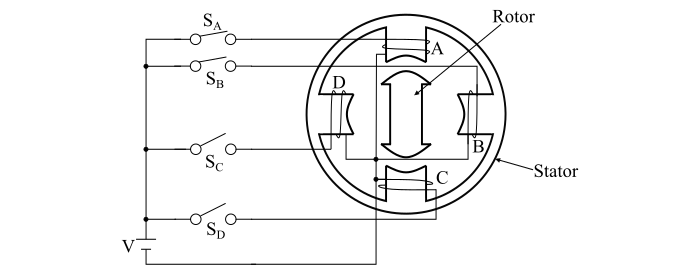# What is Single-Stack Variable Reluctance Stepper Motor?

The working principle of a single-stack variable reluctance (VR) stepper motor is based on the property of the flux lines to occupy the low reluctance path. Therefore, in a variable reluctance stepper motor, the stator and rotor get aligned such that the magnetic reluctance is minimum.

## Construction of Single-Stack Variable Reluctance Stepper Motor

A single-stack variable reluctance stepper motor consists of a salient pole stator. The stator has concentrated windings placed over the stator poles. The number of stator phases depends upon the connection of stator coils. The rotor of the single-stack variable reluctance stepper motor is a slotted structure made up of ferromagnetic material and carries no winding.

In a single-stack variable reluctance stepper motor, both the stator and rotor are made up of high quality magnetic materials having very high permeability so that the excitation current required by the motor is very small.## Operation of Single-stack Variable Reluctance Stepper Motor

When the stator phases are excited in a proper sequence from a DC source with the help of semiconductor switches, a magnetic field is produced in the motor. The ferromagnetic rotor occupies the position which presents minimum reluctance in the path of stator field. Thus, the rotor axis aligns itself to the axis of stator magnetic field.

Consider a single-stack variable reluctance stepper motor has 4-phases, 4/2-pole (i.e., 4-poles in the stator and 2-poles in the rotor). The 4-phases viz. A, B, C, D of the motor are connected to a DC source through the semiconductor switches SA, SB, SC and SD respectively. The stator phase windings of the motor are excited in the sequence A, B, C, D, A.

When the phase winding A is excited, the rotor aligns with the axis of the phase A. The rotor will remain stable in this position and cannot move until the phase A is de-energised.

Next, the phase B is energised and the phase A is de-energised. The rotor will move through 90° in the clockwise direction to align with the stator field which now lies along the axis of phase B.

Now, the phase C is excited and the phase B is de-energised, the rotor moves through a further step of 90° in the clockwise direction. In this position, the rotor aligns with the magnetic field of the stator which now lies along the axis of the phase C. Hence, as the phases are excited in the sequence A, B, C, D, A the rotor moves through a step of 90° at each transition in the clockwise direction. The rotor completes one revolution through four steps. The direction of the rotation of the rotor can be reversed by reversing the sequence of switching the windings i.e. for the rotation in the anticlockwise direction, the sequence of the phase excitation would be A, D, C, B, A.

From the above discussion, it is clear that the direction of the rotation of the rotor of a single-stack variable reluctance stepper motor depends only on the sequence of switching the phases and is independent of the direction of currents through the phases.

The magnitude of the step angle for any variable reluctance stepper motor is given by

$$\mathrm{Step\:angle,\:α =\frac{360°}{𝑚_{𝑠}\:𝑁_{𝑟}}}$$

Where,

• ms is the number of stator phases

• Nr is the number of rotor poles.

If Ns being the number stator poles, then the step angle of the variable reluctance stepper motor can also be expressed as

$$\mathrm{Step\:angle,\:α =\frac{(𝑁_{𝑠} − 𝑁_{𝑟} ) \times 360°}{𝑁_{𝑠}\:𝑁_{𝑟}}}$$

The step angle of a variable reluctance stepper motor can be reduced from 90° to 45° by exciting the phases in the sequence A, A+B, B, B+C, C, C+D, D, D+A, A. Here (A+B) means the phases A and B are excited together and hence the resultant stator magnetic field will be midway between the poles carrying the phase windings A and B, i.e., the resultant field axis makes an angle of 45° with axis of the pole A in the clockwise direction.

Therefore, when phase A is excited, the rotor aligns with the axis of the phase A. When the phases A and B are excited together, the rotor moves by 45° in the clockwise direction. Thus, this method of gradually shifting of excitation from one phase to another with an intermediate step is known as micro-stepping. The micro-stepping is used to realise smaller steps. In order to obtain a lower value of step angle, more number of poles on the stator and teeth on the rotor are used.